# Transfinite induction

(diff) ← Older revision | Latest revision (diff) | Newer revision → (diff)
A principle enabling one to infer thatholds for every elementof a well-ordered classif it is established that for each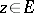the truth offor allimplies the truth of: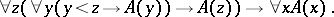Whenis the segment of ordinal numbers less than(cf. Ordinal number), the following is an equivalent formulation: If,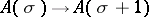and ifis preserved under limits, i.e.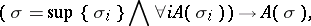then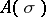for any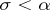. A special case of transfinite induction is mathematical induction. If the relationdefines on the classa well-founded tree (i.e. a tree all branches of which terminate), then transfinite induction for such anis equivalent to bar induction: Ifis true for all end vertices and is inherited on moving from them towards the root, thenis true for the root. This form is important in intuitionistic mathematics. The deductive power of a formal system can often be measured in terms of provability of transfinite induction up to various ordinals.